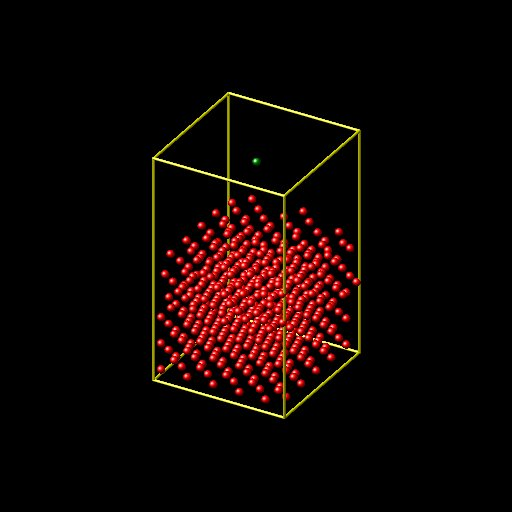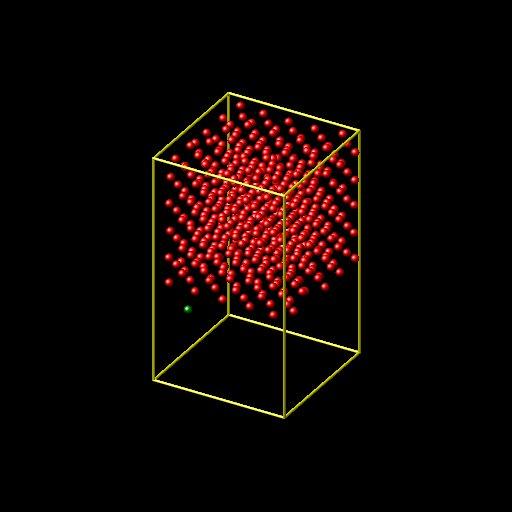# Nickel Surface is Moving Up when i run "fix nve" over long iterations

Hi All,

I am trying to calculate the heat exchange between Ni surface and
Argon atom, but the surface is moving up if i run the simulation long
time. I attached the initial and final positions of Nickel surfaces
and my input and log files. I would appreciate if someone can help me
with this issue.

Regards,
Emmain.NiArInteraction_501 (1.57 KB)

log.lammps (76.1 KB)

the velocities are set random, so maybe you have a drift from the center of mass velocity (the flying ice cube)? try setting velocity of mobile group to zero:

“The zero style adjusts the velocities of the group of atoms so that the aggregate linear or angular momentum is zero. No other changes are made to the velocities of the atoms. If the rigid option is specified (see below), then the zeroing is performed on individual rigid bodies, as defined by the fix rigid or fix rigid/small commands. In other words, zero linear will set the linear momentum of each rigid body to zero, and zero angular will set the angular momentum of each rigid body to zero. This is done by adjusting the velocities of the atoms in each rigid body.”

http://lammps.sandia.gov/doc/velocity.html